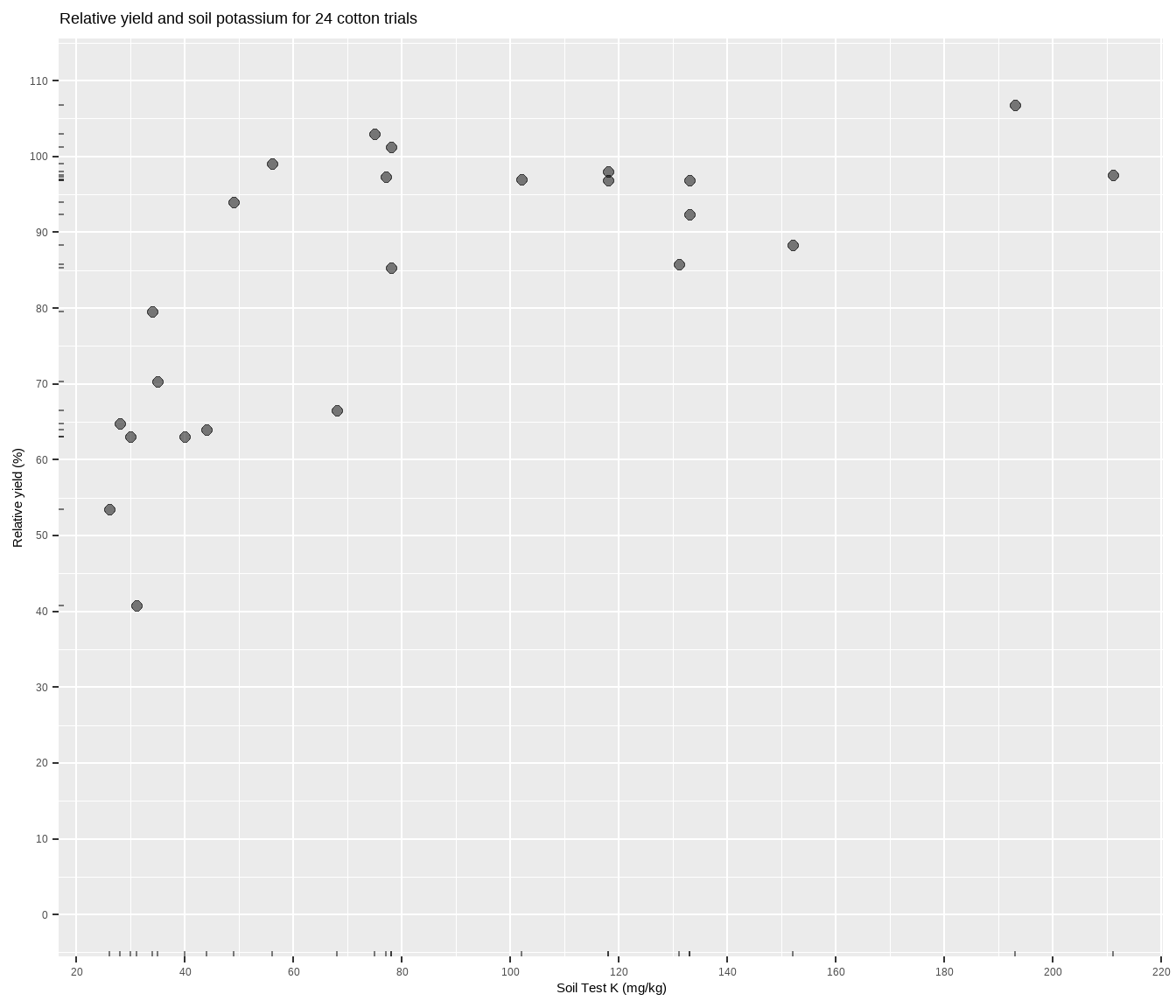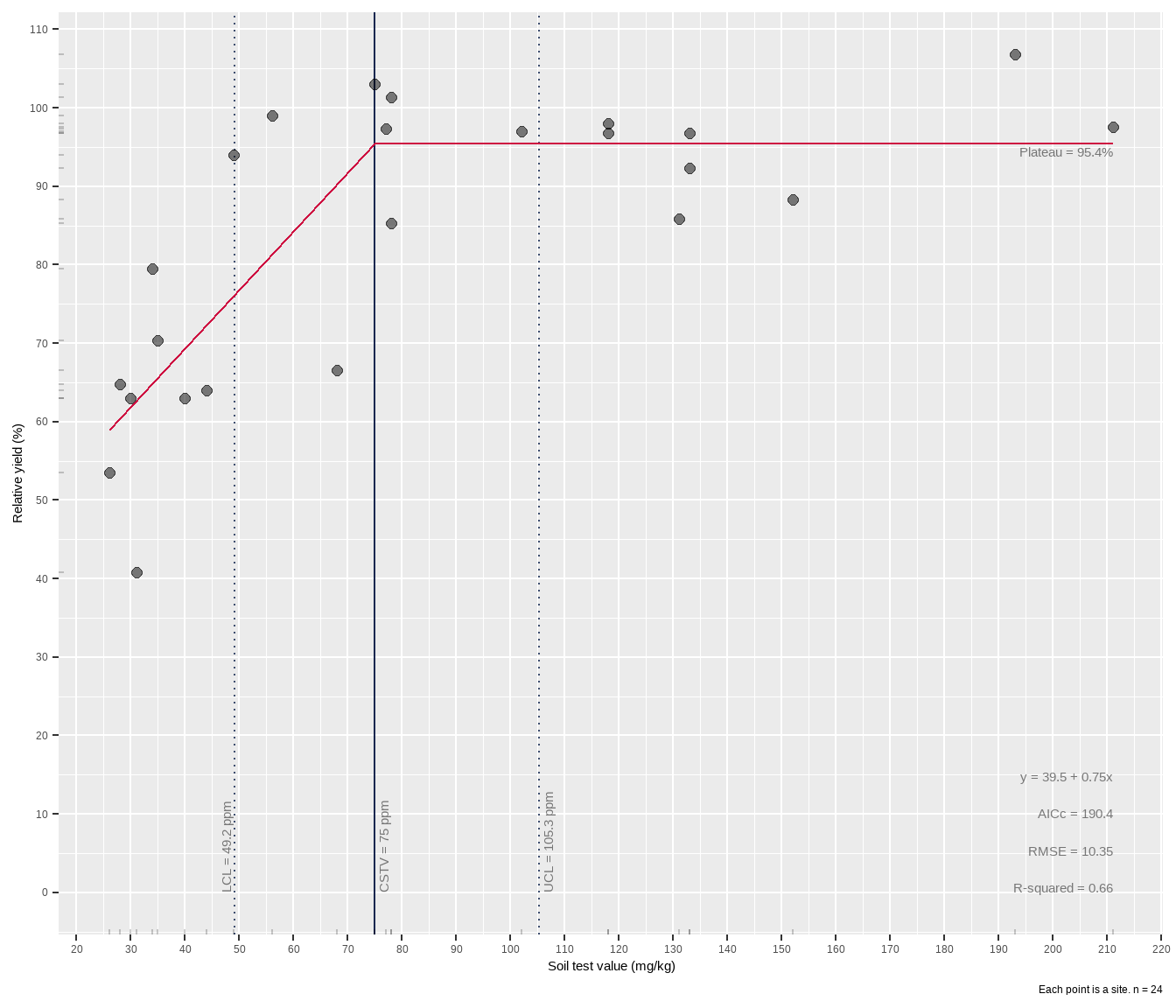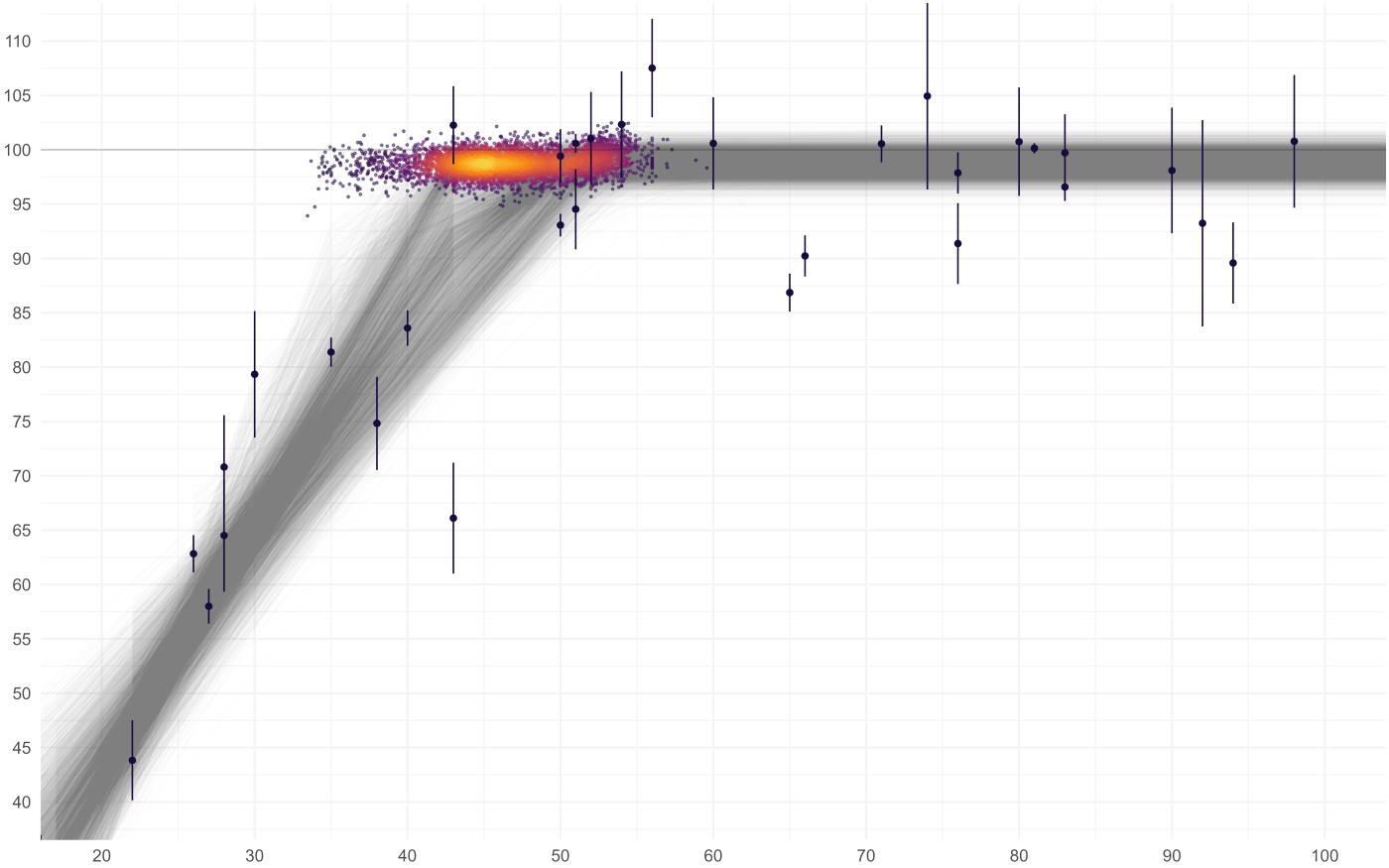# Bootstrap confidence intervals on a linear-plateau model in R

Crop yields go up a line, then level off at some uncertain point.
agriculture
statistics
modeling
soil chemistry
soil testing
soiltestcorr
R
Published

July 26, 2022

Modified

July 26, 2022

This post is about estimating uncertainty on a nonlinear model parameter or outcome. In the context of soil test correlation, this parameter or model outcome could represent a critical soil test value.

My approach is based on bootstrap confidence intervals, which has a tidyverse accent. You can also read about bootstraps from the nlraa package.

``````library(agridat)   # for the data
library(tidyverse) # for data manipulation and plotting and so on
library(nlraa)     # for self-starting models``````
``Warning: S3 method 'print.boot' was declared in NAMESPACE but not found``
``````library(minpack.lm)# for nls with Levenberg-Marquardt algorithm
library(rsample)   # for bootstraps
library(nlstools)  # for residual plots
library(modelr)    # for the r-squared and rmse
library(devtools)  # for sourcing the lin_plateau() function in Method 3``````

## The Data

The dataset I’ll use can be accessed through the `agridat` package. In this soil test correlation dataset, more potassium measured in the soil should correlate to more cotton yield, but the relationship will most likely not be linear. Let’s plot it to see what we’re working with.

``````cotton <- tibble(x = agridat::cate.potassium\$potassium,
y = agridat::cate.potassium\$yield)

cotton %>%
ggplot(aes(x, y)) +
geom_point(size = 2, alpha = 0.5) +
geom_rug(alpha = 0.5, length = unit(2, "pt")) +
scale_x_continuous(breaks = seq(0, 1000, 20)) +
scale_y_continuous(limits = c(0, 110),
breaks = seq(0, max(cotton\$y) * 2, 10)) +
labs(title = paste(
"Relative yield and soil potassium for", nrow(cotton), "cotton trials"),
y = "Relative yield (%)",
x = "Soil Test K (mg/kg)")``````The relationship between soil test potassium (STK) and relative cotton yield is not linear. Perhaps a polynomial function could be fit, or the data could be transformed, but we’ll fit a nonlinear model known as the linear-plateau (LP), or lin-plat1. Looking at the plot, notice how the relative yield of the cotton does not appear to increase anymore from about 70-200 ppm K (the plateau!). Scroll to the bottom of this page if you struggle to visualize this.

• 1 This isn’t true, but I wish it was.

• ## Basic method of fitting LP

Let’s make a function for the LP model to be used with `nls()` in base R.

``````# a = intercept
# b = slope
# cx = critical X value = join/break point
#
lp <- function(x, a, b, cx) {
if_else(
condition = x < cx,
true = a + b * x,
false = a + b * cx)
}``````

This function says that up until some join point, the relationship is linear, after which the stick breaks and it is a flat line (plateau). Using the `nls()` function in base R requires that we provide starting values for each of the model parameters. Starting values are critical, and the computer doesn’t really know where to start, so poor starting values may result in failures of the model to converge as the algorithms working their tails off behind the scenes try to home in on the best values. It helps, though, to keep in mind that with this sort of data we can expect:

1. the intercept will not be greater than the maximum Y value
2. the slope will be positive
3. the join point will not be less than your minimum X value (and, ideally, will be less than than your maximum2).
• 2 If the join point is equal to or greater than your maximum X value, then you have not fitted a LP model. You have fitted a regular, ol’ fashioned, straight line.

• ``````fit <- nls(formula = y ~ lp(x, a, b, jp),
data = cotton,
start = list(a = 30,
b = 1,
jp = 70))``````
``Error in nls(formula = y ~ lp(x, a, b, jp), data = cotton, start = list(a = 30, : step factor 0.000488281 reduced below 'minFactor' of 0.000976562``
``summary(fit)``
``Error in summary(fit): object 'fit' not found``

And it failed3. If we try running this again but include `trace = TRUE` as an additional argument, we see that `nls()` got close to finishing, but it could be it failed to converge because the data is too noisy/gappy around the values we are guessing the join point.

• 3 What if the starting values result in a failure of converge? Check out these tips from Dr. Miguez.

• Before trying another method to get starting values, let’s try `nlsLM()` first from the `minpack.lm` package, which uses a different algorithm.

``````LP_mod <- minpack.lm::nlsLM(formula = y ~ lp(x, a, b, cx),
data = cotton,
start = list(a = 30,
b = 1,
cx = 70))

summary(LP_mod)``````
``````
Formula: y ~ lp(x, a, b, cx)

Parameters:
Estimate Std. Error t value Pr(>|t|)
a   39.8388     9.4844   4.200 0.000402 ***
b    0.7408     0.2077   3.567 0.001822 **
cx  75.0000    10.8467   6.915 7.85e-07 ***
---
Signif. codes:  0 '***' 0.001 '**' 0.01 '*' 0.05 '.' 0.1 ' ' 1

Residual standard error: 11.06 on 21 degrees of freedom

Number of iterations to convergence: 17
Achieved convergence tolerance: 1.49e-08``````

Worked fine. Now to take this and put it into a framework for bootstrapping the tidy way. First, make a function that can fit the LP on the analysis portion of the data split created with the `rsample` package. Then create the bootstraps, and fit the LP model to each. But what if a model fails? The `purrr` package includes `possibly()` which I’ve used to produce a NULL result if the `nls()` step fails to converge.

``````fit_LP <- function(split) {
fit <- nlsLM(formula = y ~ lp(x, a, b, cx),
data = analysis(split),
start = as.list(coef(LP_mod)))

return(fit)
}

# Must set seed to reproduce the same results every time
set.seed(911)

lp_bootstraps <- cotton %>%
bootstraps(times = 100) %>%
mutate(models = map(splits, possibly(fit_LP, otherwise = NULL)))

lp_bootstraps``````
``````# Bootstrap sampling
# A tibble: 100 × 3
splits          id           models
<list>          <chr>        <list>
1 <split [24/9]>  Bootstrap001 <nls>
2 <split [24/9]>  Bootstrap002 <nls>
3 <split [24/8]>  Bootstrap003 <nls>
4 <split [24/9]>  Bootstrap004 <nls>
5 <split [24/10]> Bootstrap005 <nls>
6 <split [24/8]>  Bootstrap006 <nls>
9 <split [24/9]>  Bootstrap009 <nls>
10 <split [24/8]>  Bootstrap010 <nls>
# … with 90 more rows``````

Next, we have two pathways to getting the 95% confidence interval for the critical X parameter (`cx`). For each, we’ll need to get the model coefficients with `broom::tidy()`. Then we can `unnest` the coefs, pivot, and calculate the CI with `quantile()`, OR we can skip the unnesting and use the nifty interval percentile function `int_pctl()` from `rsample`.

``````param_table <- lp_bootstraps %>%
mutate(coefs = map(models, tidy)) %>%
unnest(coefs) %>%
select(-(std.error:p.value)) %>%
pivot_wider(names_from = term,
values_from = estimate)

param_table``````
``````# A tibble: 100 × 6
splits          id           models      a     b    cx
<list>          <chr>        <list>  <dbl> <dbl> <dbl>
1 <split [24/9]>  Bootstrap001 <nls>  -145.  6.52   36.5
2 <split [24/9]>  Bootstrap002 <nls>    49.6 0.617  75.0
3 <split [24/8]>  Bootstrap003 <nls>    51.2 0.455  94.0
4 <split [24/9]>  Bootstrap004 <nls>    35.8 0.671  89.0
5 <split [24/10]> Bootstrap005 <nls>    45.6 0.618  80.3
6 <split [24/8]>  Bootstrap006 <nls>    34.0 0.944  65.2
7 <split [24/7]>  Bootstrap007 <nls>    39.7 0.763  75.0
8 <split [24/7]>  Bootstrap008 <nls>    42.6 0.597  90.5
9 <split [24/9]>  Bootstrap009 <nls>    32.0 0.826  75.0
10 <split [24/8]>  Bootstrap010 <nls>    36.0 0.909  64.0
# … with 90 more rows``````
``quantile(param_table\$cx, c(0.025, 0.975), na.rm = TRUE)``
``````     2.5%     97.5%
50.00496 103.73207 ``````

And using `rsample`:

``````lp_bootstraps %>%
mutate(coefs = map(models, tidy)) %>%
int_pctl(coefs)``````
``````Warning: Recommend at least 1000 non-missing bootstrap resamples for terms: `a`,
`b`, `cx`.``````
``````# A tibble: 3 × 6
term  .lower .estimate .upper .alpha .method
<chr>  <dbl>     <dbl>  <dbl>  <dbl> <chr>
1 a     -6.37      30.3   57.1    0.05 percentile
2 b      0.398      1.01   1.88   0.05 percentile
3 cx    50.0       71.8  104.     0.05 percentile``````

Pretty cool. Did you notice the warning message? With bootstrap resampling techniques, more is better (at least to a point, and depending on your patience). The 1000 mentioned in the warning message is a standard recommendation; my code only used 100. So let’s see if/how our 95% confidence intervals change if we do….2000!

``````set.seed(911)

cotton %>%
bootstraps(times = 2000) %>%
mutate(models = map(splits, possibly(fit_LP, otherwise = NULL)),
coefs = map(models, tidy)) %>%
int_pctl(coefs)``````
``````# A tibble: 3 × 6
term  .lower .estimate .upper .alpha .method
<chr>  <dbl>     <dbl>  <dbl>  <dbl> <chr>
1 a     -9.26      29.7   55.2    0.05 percentile
2 b      0.426      1.02   2.15   0.05 percentile
3 cx    49.0       71.4  102.     0.05 percentile``````

So the confidence intervals for the `cx` parameter didn’t change much.

# Bootstrap confidence intervals with a plot!

I’ve added these bootstrap steps into the `lin_plateau()` function described in my other post. Let’s get that function.

``````devtools::source_url("https://raw.githubusercontent.com/austinwpearce/SoilTestCocaCola/main/lin_plateau.R")

# run `lin_plateau` without parentheses in R console to see source code``````

By using the `confint = TRUE` argument, we can get an estimate of the uncertainty around the join point parameter of the linear-plateau. As a default, only 500 bootstrap replicates (`boot_R`) are used, for a quick estimate of the 95% CI. Bump that up to 1000 or more at your pleasure.

``lin_plateau(cotton, x, y, confint = TRUE)``
``````Warning: Recommend at least 1000 non-missing bootstrap resamples for terms: `a`,
`b`, `cx`.``````
``````# A tibble: 1 × 11
intercept slope equation   cstv   lcl   ucl plateau  AICc  rmse rsqua…¹ boot_R
<dbl> <dbl> <chr>     <dbl> <dbl> <dbl>   <dbl> <dbl> <dbl>   <dbl>  <dbl>
1      39.5  0.75 39.5 + 0…    75  49.2  105.    95.4  190.  10.4    0.66    500
# … with abbreviated variable name ¹​rsquared``````
``lin_plateau(cotton, x, y, confint = TRUE, plot = TRUE)``
``````Warning: Recommend at least 1000 non-missing bootstrap resamples for terms: `a`,
`b`, `cx`.``````The critical value for soil test K appears to be 75 mg kg-1 K, with a 95% CI from about 49 to 105 mg kg-1 K. Now why do we go to all this trouble with bootstrap confidence intervals? Because we cannot assume that the uncertainty around the join point is normally distributed. This could be visualized by plotting all the join points from all the bootstraps (example from another dataset below). With nonlinear models, the distribution of a parameter is often not normal.Visualization of the linear-plateau break point on another dataset showing the variation estimated by thousands of bootstraps.